# Feature extraction¶

The term Feature Extraction refers to techniques aiming at extracting added value information from images. These extracted items named features can be local statistical moments, edges, radiometric indices, morphological and textural properties. For example, such features can be used as input data for other image processing methods like Segmentation and Classification .

## Local statistics extraction¶

This application computes the 4 local statistical moments on every pixel in the selected channel of the input image, over a specified neighborhood. The output image is multi band with one statistical moment (feature) per band. Thus, the 4 output features are the Mean, the Variance, the Skewness and the Kurtosis. They are provided in this exact order in the output image.

The LocalStatisticExtraction application has the following input parameters:

--in the input image to compute the features on

--channel the selected channel index in the input image to be

processed (default value is 1)

--radius the computational window radius (default value is 3

pixels)

--out the output image containing the local statistical moments

The application can be used like this:

otbcli_LocalStatisticExtraction  -in        InputImage.tif
-channel   1
-out       OutputImage.tif


## Edge extraction¶

This application Computes edge features on every pixel in the selected channel of the input image.

The EdgeExtraction application has the following input parameters:

--in the input image to compute the features on

--channel the selected channel index in the input image to be

processed (default value is 1)

• -filter the choice of edge detection method (gradient/sobel/touzi) (default value is gradient)

-(-filter.touzi.xradius) the X Radius of the Touzi processing neighborhood (only if filter==touzi) (default value is 1 pixel) __

• (-filter.touzi.yradius) the Y Radius of the Touzi processing neighborhood (only if filter==touzi) (default value is 1 pixel)

--out the output mono band image containing the edge features

The application can be used like this:

otbcli_EdgeExtraction  -in        InputImage
-channel   1
-filter    sobel
-out       OutputImage


or like this if filter==touzi:

otbcli_EdgeExtraction  -in                    InputImage
-channel               1
-filter                touzi
-out                   OutputImage


This application computes radiometric indices using the channels of the input image. The output is a multi band image into which each channel is one of the selected indices.

The RadiometricIndices application has the following input parameters:

--in the input image to compute the features on

--out the output image containing the radiometric indices

--channels.blue the Blue channel index in the input image (default

value is 1)

--channels.green the Green channel index in the input image

(default value is 1)

--channels.red the Red channel index in the input image (default

value is 1)

--channels.nir the Near Infrared channel index in the input image

(default value is 1)

--channels.mir the Mid-Infrared channel index in the input image

(default value is 1)

--list the list of available radiometric indices (default value is

Vegetation:NDVI)

Note that band numbering starts at 1.

The available radiometric indices to be listed into -list with their relevant channels in brackets are:

Index

Description

Required bands

Vegetation:NDVI

Normalized difference vegetation index

Red, NIR

Vegetation:TNDVI

Transformed normalized difference vegetation index

Red, NIR

Vegetation:RVI

Ratio vegetation index

Red, NIR

Vegetation:SAVI

Red, NIR

Vegetation:TSAVI

Red, NIR

Vegetation:MSAVI

Red, NIR

Vegetation:MSAVI2

Modified soil adjusted vegetation index 2

Red, NIR

Vegetation:GEMI

Global environment monitoring index

Red, NIR

Vegetation:IPVI

Infrared percentage vegetation index

Red, NIR

Vegetation:LAIFromNDVILog

Leaf Area Index from log NDVI

Red, NIR

Vegetation::LAIFromReflLinear

Leaf Area Index from reflectances with linear combination

Red, NIR

Vegetation::LAIFromNDVIFormo

Leaf Area Index from Formosat 2 TOC

Red, NIR

Water:NDWI

Normalized difference water index (Gao 1996)

NIR, MIR

Water:NDWI2

Normalized difference water index (Mc Feeters 1996)

Green, NIR

Water:MNDWI

Modified normalized difference water index (Xu 2006)

Green, MIR

Water:NDTI

Normalized difference turbidity index (Lacaux et al.)

Green, Red

Soil:RI

Redness index

Green, Red

Soil:CI

Color index

Green, Red

Soil:BI

Brightness index

Green, Red

Soil:BI2

Brightness index 2

Green, Red, NIR

BuiltUp:ISU

Built Surfaces Index

Red, NIR

The application can be used as follows, which would produce an output image containing 3 bands, respectively with the Vegetation:NDVI, Vegetation:RVI and Vegetation:IPVI radiometric indices in this exact order:

otbcli_RadiometricIndices -in             InputImage
-out            OutputImage
-channels.red   3
-channels.green 2
-channels.nir   4
-list           Vegetation:NDVI Vegetation:RVI
Vegetation:IPVI


or as follows, which would produce a single band output image with the Water:NDWI2 radiometric index:

otbcli_RadiometricIndices -in             InputImage
-out            OutputImage
-channels.red   3
-channels.green 2
-channels.nir   4
-list           Water:NDWI2


## Morphological features extraction¶

Morphological features can be highlighted by using image filters based on mathematical morphology either on binary or gray scale images.

### Binary morphological operations¶

This application performs binary morphological operations (dilation, erosion, opening and closing) on a mono band image with a specific structuring element (a ball or a cross) having one radius along X and another one along Y. NB: the cross shaped structuring element has a fixed radius equal to 1 pixel in both X and Y directions.

The BinaryMorphologicalOperation application has the following input parameters:

--in the input image to be filtered

--channel the selected channel index in the input image to be

processed (default value is 1)

--structype the choice of the structuring element type

(ball/cross) (default value is ball)

-(-xradius) the ball structuring element X Radius

(default value is 5 pixels)

-(-yradius) the ball structuring element Y Radius

(default value is 5 pixels)

--filter the choice of the morphological operation

(dilate/erode/opening/closing) (default value is dilate)

-(-foreval) the foreground value

(Used for dilate/erode/opening/closing morphological operations) (default value is 1)

-(-backval) the background value

(Used for dilate/erode/opening morphological operations) (default value is 0)

--out the output filtered image

The application can be used as follows:

otbcli_BinaryMorphologicalOperation  -in                     InputImage
-channel                1
-structype              ball
-filter                 opening
-foreval                1.0
-backval                0.0
-out                    OutputImage


### Gray scale morphological operations¶

This application performs morphological operations (dilation, erosion, opening and closing) on a gray scale mono band image with a specific structuring element (a ball or a cross) having one radius along X and another one along Y. NB: the cross shaped structuring element has a fixed radius equal to 1 pixel in both X and Y directions.

The GrayScaleMorphologicalOperation application has the following input parameters:

--in the input image to be filtered

--channel the selected channel index in the input image to be

processed (default value is 1)

--structype the choice of the structuring element type

(ball/cross) (default value is ball)

-(-structype.ball.xradius) the ball structuring element X Radius

(only if structype==ball) (default value is 5 pixels)

-(-structype.ball.yradius) the ball structuring element Y Radius

(only if structype==ball) (default value is 5 pixels)

--filter the choice of the morphological operation

(dilate/erode/opening/closing) (default value is dilate)

--out the output filtered image

The application can be used as follows:

otbcli_GrayScaleMorphologicalOperation  -in                     InputImage
-channel                1
-structype              ball
-filter                 opening
-out                    OutputImage


## Textural features extraction¶

Texture features can be extracted with the help of image filters based on texture analysis methods like Haralick and structural feature set (SFS).

### Haralick texture features¶

This application computes Haralick, advanced and higher order texture features on every pixel in the selected channel of the input image. The output image is multi band with a feature per band.

The HaralickTextureExtraction application has the following input parameters:

--in the input image to compute the features on

--channel the selected channel index in the input image to be

processed (default value is 1)

--texture the texture set selection [simple/advanced/higher]

(default value is simple)

--parameters.min the input image minimum (default value is 0)

--parameters.max the input image maximum (default value is 255)

--parameters.xrad the X Radius of the processing neighborhood

(default value is 2 pixels)

--parameters.yrad the Y Radius of the processing neighborhood

(default value is 2 pixels)

--parameters.xoff the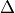X Offset for the

co-occurrence computation (default value is 1 pixel)

--parameters.yoff theY Offset for the

co-occurrence computation (default value is 1 pixel)

--parameters.nbbin the number of bin per axis for histogram

generation (default value is 8)

--out the output multi band image containing the selected texture

features (one feature per band)

The available values for -texture with their relevant features are:

--texture=simple: In this case, 8 local Haralick textures features

will be processed. The 8 output image channels are: Energy, Entropy, Correlation, Inverse Difference Moment, Inertia, Cluster Shade, Cluster Prominence and Haralick Correlation. They are provided in this exact order in the output image. Thus, this application computes the following Haralick textures over a neighborhood with user defined radius. To improve the speed of computation, a variant of Grey Level Co-occurrence Matrix(GLCM) called Grey Level Co-occurrence Indexed List (GLCIL) is used. Given below is the mathematical explanation on the computation of each textures. Here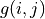is the frequency of element in the GLCIL whose index is i, j. GLCIL stores a pair of frequency of two pixels taken from the given offset and the cell index (i, j) of the pixel in the neighborhood window. :(where each element in GLCIL is a pair of pixel index and it’s frequency,is the frequency value of the pair having index is i, j).

“Energy”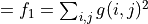“Entropy”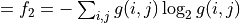, or 0 if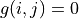“Correlation”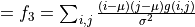“Inverse Difference Moment”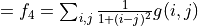“Inertia”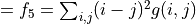(sometimes called “contrast”)

“Cluster Shade”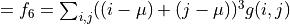“Cluster Prominence”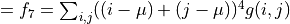“Haralick’s Correlation”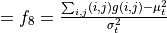where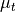and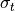are the mean and standard deviation of the row (or column, due to symmetry) sums. Above,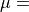(weighted pixel average)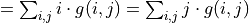(due to matrix symmetry), and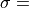(weighted pixel variance)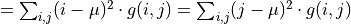(due to matrix symmetry).

--texture=advanced: In this case, 10 advanced texture features

will be processed. The 10 output image channels are: Mean, Variance, Dissimilarity, Sum Average, Sum Variance, Sum Entropy, Difference of Entropies, Difference of Variances, IC1 and IC2. They are provided in this exact order in the output image. The textures are computed over a sliding window with user defined radius.

To improve the speed of computation, a variant of Grey Level Co-occurrence Matrix(GLCM) called Grey Level Co-occurrence Indexed List (GLCIL) is used. Given below is the mathematical explanation on the computation of each textures. Hereis the frequency of element in the GLCIL whose index is i, j. GLCIL stores a pair of frequency of two pixels taken from the given offset and the cell index (i, j) of the pixel in the neighborhood window. :(where each element in GLCIL is a pair of pixel index and it’s frequency,is the frequency value of the pair having index is i, j).

“Mean”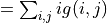“Sum of squares: Variance”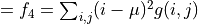“Dissimilarity”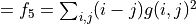“Sum average”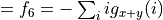“Sum Variance”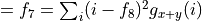“Sum Entropy”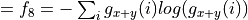“Difference variance”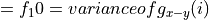“Difference entropy”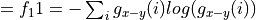“Information Measures of Correlation IC1”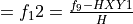“Information Measures of Correlation IC2”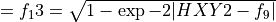Above,(weighted pixel average)(due to matrix summetry), and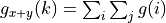where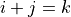and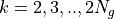and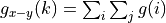where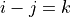and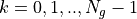--texture=higher: In this case, 11 local higher order statistics

texture coefficients based on the grey level run-length matrix will be processed. The 11 output image channels are: Short Run Emphasis, Long Run Emphasis, Grey-Level Nonuniformity, Run Length Nonuniformity, Run Percentage, Low Grey-Level Run Emphasis, High Grey-Level Run Emphasis, Short Run Low Grey-Level Emphasis, Short Run High Grey-Level Emphasis, Long Run Low Grey-Level Emphasis and Long Run High Grey-Level Emphasis. They are provided in this exact order in the output image. Thus, this application computes the following Haralick textures over a sliding window with user defined radius: (where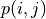is the element in cell i, j of a normalized Run Length Matrix,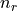is the total number of runs and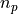is the total number of pixels):

“Short Run Emphasis”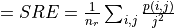“Long Run Emphasis”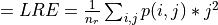“Grey-Level Nonuniformity”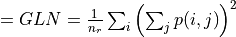“Run Length Nonuniformity”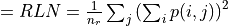“Run Percentage”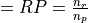“Low Grey-Level Run Emphasis”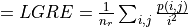“High Grey-Level Run Emphasis”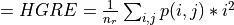“Short Run Low Grey-Level Emphasis”“Short Run High Grey-Level Emphasis”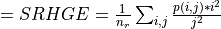“Long Run Low Grey-Level Emphasis”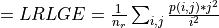“Long Run High Grey-Level Emphasis”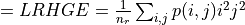The application can be used like this:

otbcli_HaralickTextureExtraction  -in             InputImage
-channel        1
-texture        simple
-parameters.min 0
-parameters.max 255
-out            OutputImage


### SFS texture extraction¶

This application computes Structural Feature Set textures on every pixel in the selected channel of the input image. The output image is multi band with a feature per band. The 6 output texture features are SFS’Length, SFS’Width, SFS’PSI, SFS’W-Mean, SFS’Ratio and SFS’SD. They are provided in this exact order in the output image.

It is based on line direction estimation and described in the following publication. Please refer to Xin Huang, Liangpei Zhang and Pingxiang Li publication, Classification and Extraction of Spatial Features in Urban Areas Using High-Resolution Multispectral Imagery. IEEE Geoscience and Remote Sensing Letters, vol. 4, n. 2, 2007, pp 260-264.

The texture is computed for each pixel using its neighborhood. User can set the spatial threshold that is the max line length, the spectral threshold that is the max difference authorized between a pixel of the line and the center pixel of the current neighborhood. The adjustment constant alpha and the ratio Maximum Consideration Number, which describes the shape contour around the central pixel, are used to compute the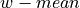value.

The SFSTextureExtraction application has the following input parameters:

--in the input image to compute the features on

--channel the selected channel index in the input image to be

processed (default value is 1)

--parameters.spethre the spectral threshold (default value is 50)

--parameters.spathre the spatial threshold (default value is 100

pixels)

--parameters.nbdir the number of directions (default value is 20)

--parameters.alpha the alpha value (default value is 1)

--parameters.maxcons the ratio Maximum Consideration Number

(default value is 5)

--out the output multi band image containing the selected texture

features (one feature per band)

The application can be used like this:

otbcli_SFSTextureExtraction -in             InputImage
-channel        1
-out            OutputImage